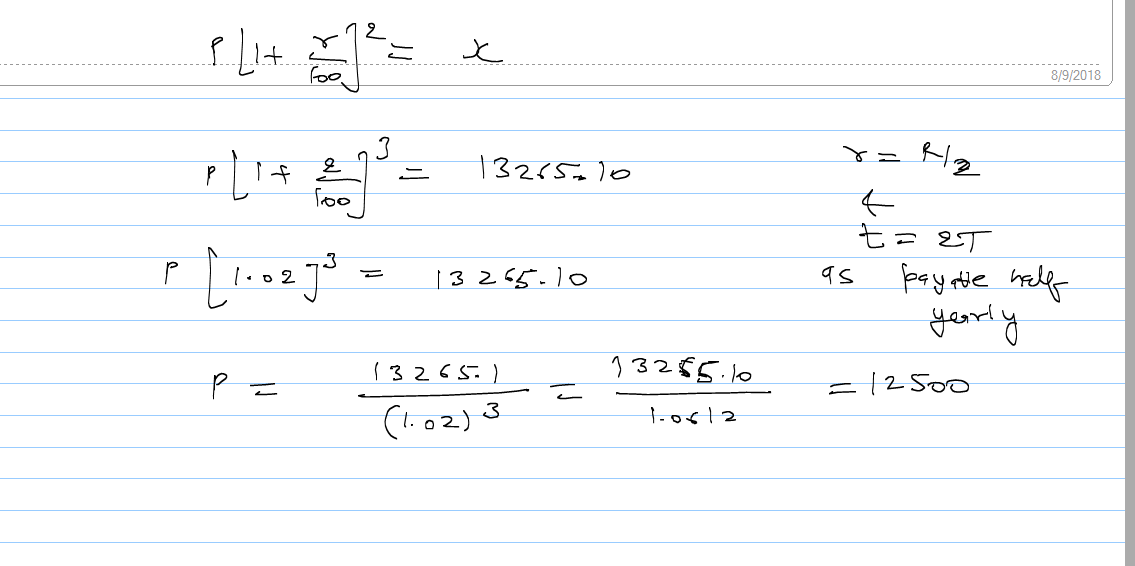# A sum put out at 4% compound interest payable half yearly  amounts to Rs 13265.10 in 11/2 years. Find the sum.Deepak Kumar Shringi
4 years ago4 years ago

The formula for annual compound interest, including principal sum, is:
A = P (1 + r/n) (nt)

Where:

A = the future value of the investment/loan, including interest
P = the principal investment amount (the initial deposit or loan amount)
r = the annual interest rate (decimal)
n = the number of times that interest is compounded per year
t = the number of years the money is invested or borrowed for

A = 13265.10 Rs.

t = 11/2 year

r = 4%

n = 2 (as, half yearly)

Therefore, 13265.10=P(1+4/(2×100))2×11/2

Or, 13265.1= P(51/50)11

Or, P=13265.1(50/51)11

Or, P = 10668.62 (approx.)

Therefore the sum of the amount was Rs. 10668.62 (approx.)